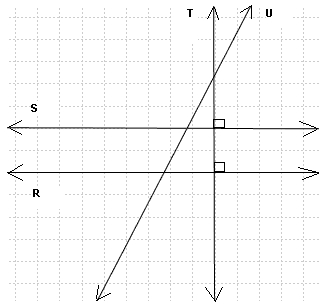True or False: Two parallel lines intersect. A triangle is a closed planar shape with 2 sides 4 sides 3 sides 5 sides A closed planar shape with 5 sides is called a pentagon hexagon square heptagon A closed planar shape with 4 sides is called a segment hexagon quadrilateral heptagon A line segment is defined by 1 point 3 points 2 points 4 points An equilateral triangle has only 2 equal sides all 3 sides equal no equal sides one right angle Which of the statements below best describes a square? A square has 4 equal sides and 4 right angles. A square has 4 equal sides. A square has 4 right angles. 2 pairs of parallel sides. Which pair of lines are parallel?. Which of these shapes are quadrilaterals? triangles? squares?. Which of these shapes are right triangles? rectangles?. Which pair of lines are parallel? perpendicular?. Find the perimeter and area of each shape.. Find the perimeter and area of each shape.. Which of these is an isosceles triangle?. Which pair of triangles are congruent?. Point O is the center of the circle below. What do you call the segments AB? CD? OD?. Answers to the Above Questions false C A C C B A A B,E,F A,D F B D,E (S,R) (T,S) and (T,R) A: Perimeter = 20 , Area = 25 B: Perimeter = 30 , Area = 54 C: Perimeter = 24 , Area = 20 D: Perimeter = 18 , Area = 20 A: Perimeter = 32 , Area = 48 B: Perimeter = 40 , Area = 80 B A and C AB: chord CD: diameter OD: radius Primary Math (grades 4 and 5) with Free Questions and Problems With Answers Middle School Math (grades 6,7,8 and 9) with Free Questions and Problems With Answers High School Math (Grades 10, 11 and 12) - Free Questions and Problems With Answers Home Page